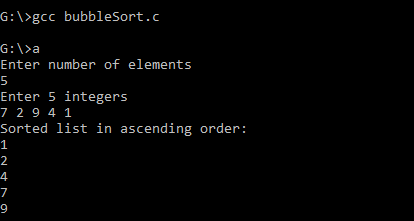Bubble Sort in C: There are many types of Data Structure. Sorting is one of them.

Bubble Sort is the most simple and easy sorting algorithm in Data Structure, as compared to other sorting techniques.

## What is Bubble Sort

Bubble Sort is an algorithm which is used to perform sorting either in ascending or descending order, by comparing the adjacent elements of the array and swap them accordingly.

## Working of Bubble Sort Algorithm​

Let’s assume that we want to sort an array arr[] in ascending order.

`arr[] = {8, 3, 6, 1};`

We will start from first 2 element, which is arr and arr. If the left element is greater than right element, we will swap them. otherwise we will leave it as it is.

Similarly, we will proceed the comparison between arr and arr, then arr and arr, and so on, till we reach the end of the array.

Now we got the largest element in the last position of array. We will again perform the same comparison from starting to get the second largest element in the second last position of array.

So, there will be n-1 number of rounds to be performed in order to sort an array, where n is the length of array.

You will understand it much better with the below explanation below.

So, to implement Bubble Sort in the array, we will perform the following steps:

In the 1st round

1. [8, 3, 6, 1] ——- [3, 8, 6, 1] //arr is compared with arr and swapped as arr is greater than arr.
2. [3, 8, 6, 1] ——- [3, 6, 8, 1] //arr is compared with arr and swapped as arr is greater than arr.
3. [3, 6, 8, 1] ——– [3, 6, 1, 8] //arr is compared with arr and swapped as arr is greater than arr.

As a result of round 1st we performed. We get a largest element which is 8, in the last position.

Now, we will perform round 2nd, to get the second largest element in the second last position.

In round 2nd

1. [3, 6, 1, 8] ——– [3, 6, 1, 8] //arr is compared with arr and no swapping is perform as arr is already smaller than arr.
2. [3, 6, 1, 8] ——– [3, 1, 6, 8] //arr is compared with arr and swapped as arr is greater than arr.

We will not compare arr and arr, as last element is already the largest element in the array.

So, here we get the second last element which is 6, in the second last position of array.

Similarly the round 3rd will be performed and we will get our sorted array in the ascending order.

## Implementation of Bubble Sort in C

```				```
#include <stdio.h>

int main()
{
int arr, n, i, j, swap;
printf("Enter number of elements\n");
scanf("%d", &n);

printf("Enter %d integers\n", n);

for (i = 0; i < n; i++)
scanf("%d", &arr[i]);

for (i = 0 ; i < n - 1; i++)
{
for (j = 0 ; j < n - i - 1; j++)
{
if (arr[j] > arr[j+1])
{
swap = arr[j];
arr[j] = arr[j+1];
arr[j+1] = swap;
}
}
}

printf("Sorted list in ascending order:\n");

for (i = 0; i < n; i++)
printf("%d\n", arr[i]);

return 0;
}
```
```

Output: